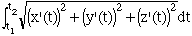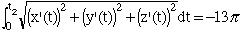Find the point on the curve r(t)=(12sint)i-(12cost)j+5tk at a distance 13pi units along the curve from the point (0,-12,0) when t=0 in the direction opposite to the direction of increasing arc length. Thanks vix Hi Vix, You have the curve expressed in vector form r(t) = x(t)i + y(t)j + z(t)k and hence you can write the arclength aoong this curve from t=t1 to t=t2 asCalculate (x'(t)) 2, (y'(t)) 2 and (z'(t)) 2 and simplify the square root of their sum. The result is a very simple expression. Since the integrand (the square root) is a positive expression, the arclength increases as t increases. The given point, (0,-12,0) results when t=0 and hence to get a decreasing arclength you can integrate from t1 = 0 to a negative value of t2. Thus evaluate the integral and solvefor t2. Harley Go to Math Central